# ML Aggarwal Class 10 Solutions for ICSE Maths Chapter 15 Circles MCQS

## ML Aggarwal Class 10 Solutions for ICSE Maths Chapter 15 Circles MCQS

ML Aggarwal Class 10 Solutions for ICSE Maths Chapter 15 Circles MCQS

Question 1.
In the given figure, O is the centre of the circle. If ∠ABC = 20°, then ∠AOC is equal to
(a) 20°
(b) 40°
(c) 60°
(d) 10°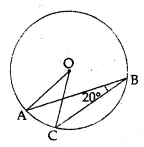Solution: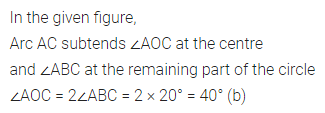Question 2.
In the given figure, AB is a diameter of the circle. If AC = BC, then ∠CAB is equal to
(a) 30°
(b) 60°
(c) 90°
(d) 45°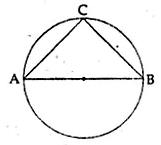Solution:Question 3.
In the given figure, if ∠DAB = 60° and ∠ABD = 50° then ∠ACB is equal to
(a) 60°
(b) 50°
(c) 70°
(d) 80°Solution: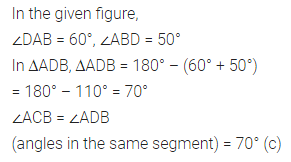Question 4.
In the given figure, O is the centre of the circle. If ∠OAB = 40°, then ∠ACB is equal to
(a) 50°
(b) 40°
(c) 60°
(d) 70°Solution: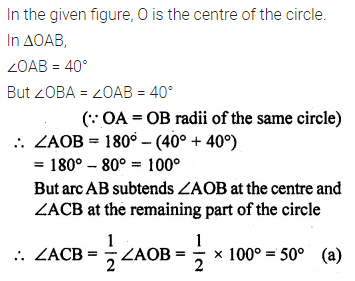Question 5.
ABCD is a cyclic quadrilateral such that AB is a diameter of the circle circumscribing it and ∠ADC = 140°, then ∠BAC is equal to
(a) 80°
(b) 50°
(c) 40°
(d) 30°
Solution:Question 6.
In the given figure, O is the centre of the circle. If ∠BAO = 60°, then ∠ADC is equal to
(a) 30°
(b) 45°
(c) 60°
(d) 120°Solution: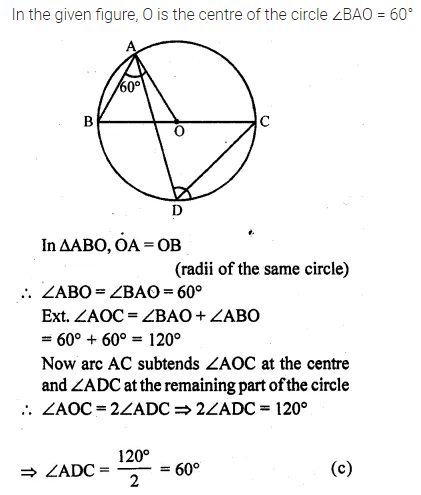Question 7.
In the given figure, O is the centre of the circle. If ∠AOB = 90° and ∠ABC = 30°, then ∠CAO is equal to
(a) 30°
(b) 45°
(c) 90°
(d) 60°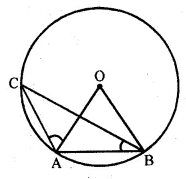Solution: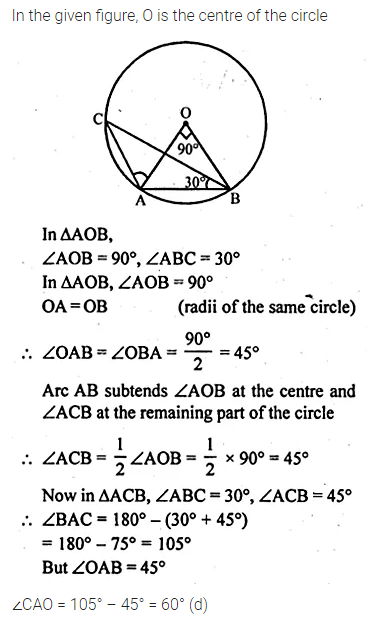Question 8.
In the given figure, O is the centre of a circle. If the length of chord PQ is equal to the radius of the circle, then ∠PRQ is
(a) 60°
(b) 45°
(c) 30°
(d) 15°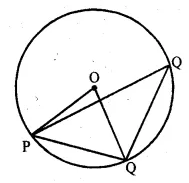Solution: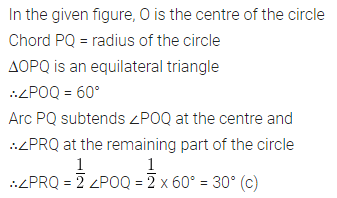Question 9.
In the given figure, if O is the centre of the circle then the value of x is
(a) 18°
(b) 20°
(c) 24°
(d) 36°Solution: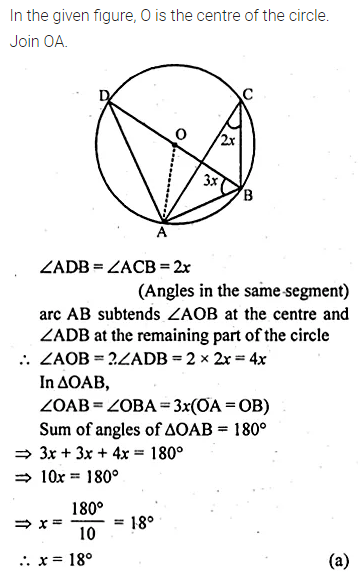Question 10.
From a point Q, the length of the tangent to a circle is 24 cm and the distance of Q from the centre is 25 cm. The radius of the circle is
(a) 7 cm
(b) 12 cm
(c) 15 cm
(d) 24.5 cm
Solution: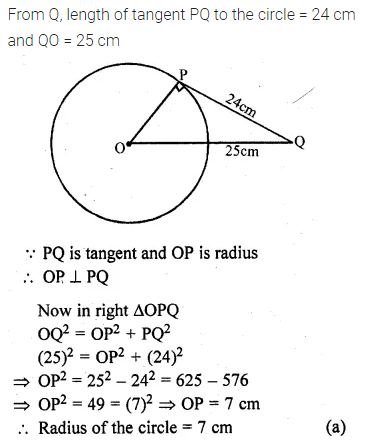Question 11.
From a point which is at a distance of 13 cm from the centre O of a circle of radius 5 cm, the pair of tangents PQ and PR to the circle are drawn. Then the area of the quadrilateral PQOR is
(a) 60 cm²
(b) 65 cm²
(c) 30 cm²
(d) 32.5 cm²
Solution: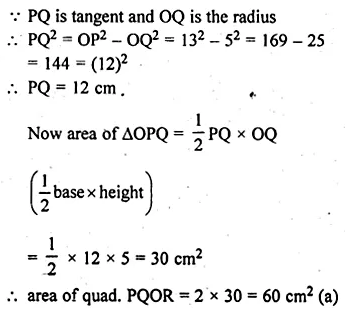Question 12.
If the angle between two radii of a circle is 130°, the angle between the tangents at the ends of the radii is
(a) 90°
(b) 50°
(c) 70°
(d) 40°
Solution: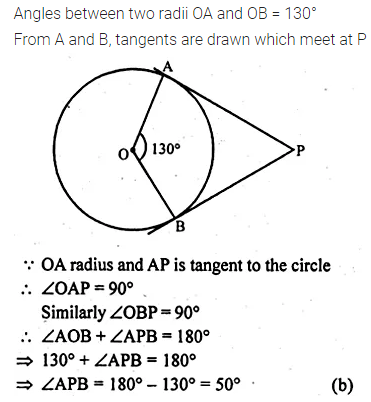Question 13.
In the given figure, PQ and PR are tangents from P to a circle with centre O. If ∠POR = 55°, then ∠QPR is
(a) 35°
(b) 55°
(c) 70°
(d) 80°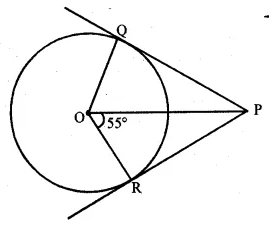Solution:Question 14.
If tangents PA and PB from an exterior point P to a circle with centre O are inclined to each other at an angle of 80°, then ∠POA is equal to
(a) 50°
(b) 60°
(c) 70°
(d) 100°
Solution:Question 15.
In the given figure, PA and PB are tangents from point P to a circle with centre O. If the radius of the circle is 5 cm and PA ⊥ PB, then the length OP is equal to
(a) 5 cm
(b) 10 cm
(c) 7.5 cm
(d) 5√2 cmSolution:Question 16.
At one end A of a diameter AB of a circle of radius 5 cm, tangent XAY is drawn to the circle. The length of the chord CD parallel to XY and at a distance 8 cm from A is
(a) 4 cm
(b) 5 cm
(c) 6 cm
(d) 8 cm
Solution:Question 17.
If radii of two concentric circles are 4 cm and 5 cm, then the length of each chord of one circle which is tangent to the other is
(a) 3 cm
(b) 6 cm
(c) 9 cm
(d) 1 cm
Solution:Question 18.
In the given figure, AB is a chord of the circle such that ∠ACB = 50°. If AT is tangent to the circle at the point A, then ∠BAT is equal to
(a) 65°
(b) 60°
(c) 50°
(d) 40°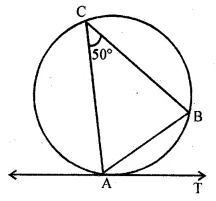Solution: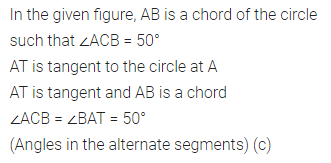Question 19.
In the given figure, O is the centre of a circle and PQ is a chord. If the tangent PR at P makes an angle of 50° with PQ, then ∠POQ is
(a) 100°
(b) 80°
(c) 90°
(d) 75°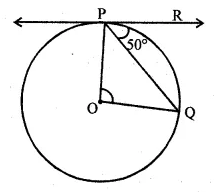Solution: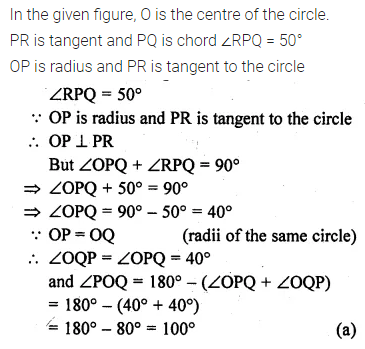Question 20.
In the given figure, PA and PB are tangents to a circle with centre O. If ∠APB = 50°, then ∠OAB is equal to
(a) 25°
(b) 30°
(c) 40°
(d) 50°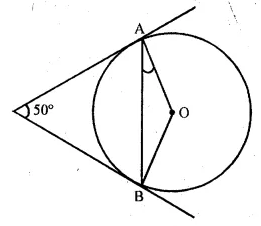Solution: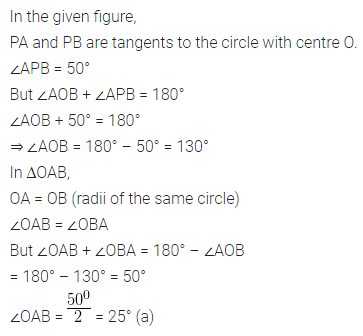Question 21.
In the given figure, sides BC, CA and AB of ∆ABC touch a circle at point D, E and F respectively. If BD = 4 cm, DC = 3 cm and CA = 8 cm, then the length of side AB is
(a) 12 cm
(b) 11 cm
(c) 10 cm
(d) 9 cmSolution:Question 22.
In the given figure, sides BC, CA and AB of ∆ABC touch a circle at the points P, Q and R respectively. If PC = 5 cm, AR = 4 cm and RB = 6 cm, then the perimeter of ∆ABC is
(a) 60 cm
(b) 45 cm
(c) 30 cm
(d) 15 cm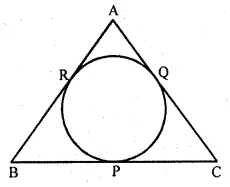Solution:Question 23.
PQ is a tangent to a circle at point P. Centre of a circle is O. If ∆OPQ is an isosceles triangle, then ∠QOP is equal to
(a) 30°
(b) 60°
(c) 45°
(d) 90°
Solution: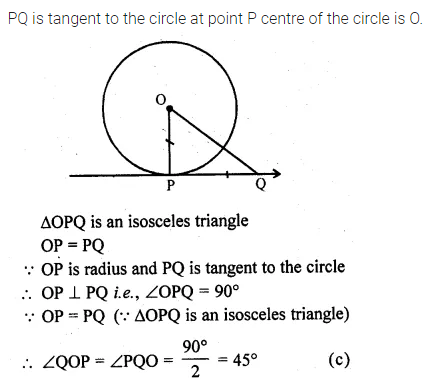Question 24.
In the given figure, PT is a tangent at T to the circle with centre O. If ∠TPO = 25°, then the value of x is
(a) 25°
(b) 65°
(c) 115°
(d) 90°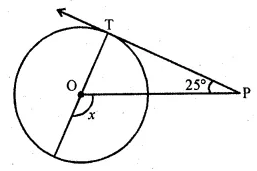Solution:Question 25.
In the given figure, PA and PB are tangents at points A and B respectively to a circle with centre O. If C is a point on the circle and ∠APB = 40°, then ∠ACB is equal to
(a) 80°
(b) 70°
(c) 90°
(d) 140°Solution: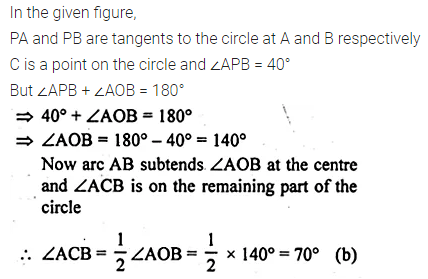Question 26.
In the given figure, two circles touch each other at A. BC and AP are common tangents to these circles. If BP = 3.8 cm, then the length of BC is equal to
(a) 7.6 cm
(b) 1.9 cm
(c) 11.4 cm
(d) 5.7 cmSolution: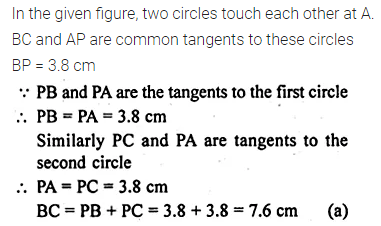Question 27.
In the given figure, if sides PQ, QR, RS and SP of a quadrilateral PQRS touch a circle at points A, B, C and D respectively, then PD + BQ is equal to
(a) PQ
(b) QR
(c) PS
(d) SRSolution: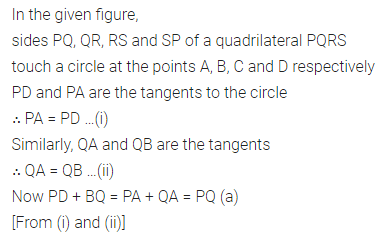Question 28.
In the given figure, PQR is a tangent at Q to a circle. If AB is a chord parallel to PR and ∠BQR = 70°, then ∠AQB is equal to
(a) 20°
(b) 40°
(b) 35°
(d) 45°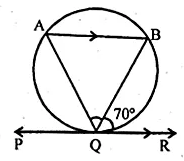Solution: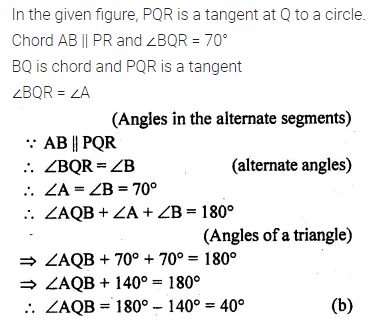Question 29.
Two chords AB and CD of a circle intersect externally at a point P. If PC = 15 cm, CD = 7 cm and AP = 12 cm, then AB is
(a) 2 cm
(b) 4 cm
(c) 6 cm
(d) none of these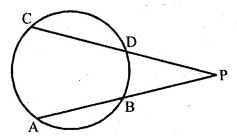Solution: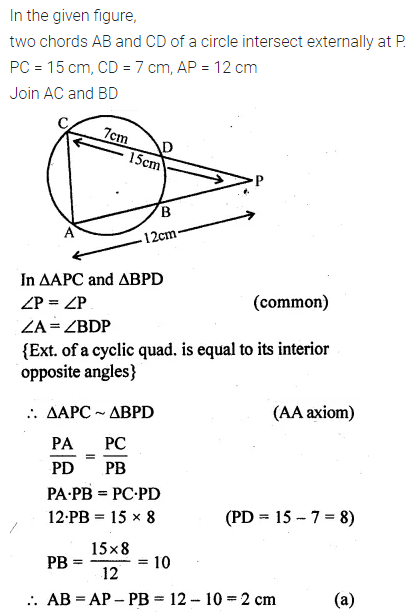ML Aggarwal Class 10 Solutions for ICSE Maths